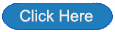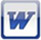#THE education site for computer science and ICT

## Starters and plenaries

##### Post-its

A fun kinesthetic activity where students work in teams to solve a puzzle.

(subscription only)

##### 30 seconds

Lesson starter

Students are shown a number of terms for exactly 30 seconds. They need to memorise as many as possible. After 30 seconds, the terms are hidden and they need to write down as many terms as they can remember along with at least one fact about that term.

(subscription only)

Lesson starter

Students become the teacher! One student is tasked with preparing a five minute revision starter about this topic for the beginning of the following lesson.

(subscription only)

##### Countdown

Lesson starter

Students are given a set of jumbled up words related to this topic, ready to be unscrambled.

(subscription only)

##### Denary to binary calculator

An Excel tool that converts denary numbers to binary##### Calculating denary numbers to binary numbers: workbook

The workbook provides students with the opportunity to calculate binary numbers from a given denary number.

it has been assumed that students have already learned how to do these calculations. This workbook is for consolidation of the techniques.

Note to teacher: it is worth going through a couple of the questions with students so that they understand how to tackle them.

(subscription only)workbook##### Calculating binary numbers to denary numbers: workbook

The workbook provides students with the opportunity to calculate denary numbers from a given binary number.

it has been assumed that students have already learned how to do these calculations. This workbook is for consolidation of the techniques.

Note to teacher: it is worth going through a couple of the questions with students so that they understand how to tackle them.

(subscription only)workbookThe workbook provides students with the opportunity to practice adding binary numbers together.

Note to teacher: it is worth going through a couple of the questions with students so that they understand how to tackle them.

(subscription only)workbook